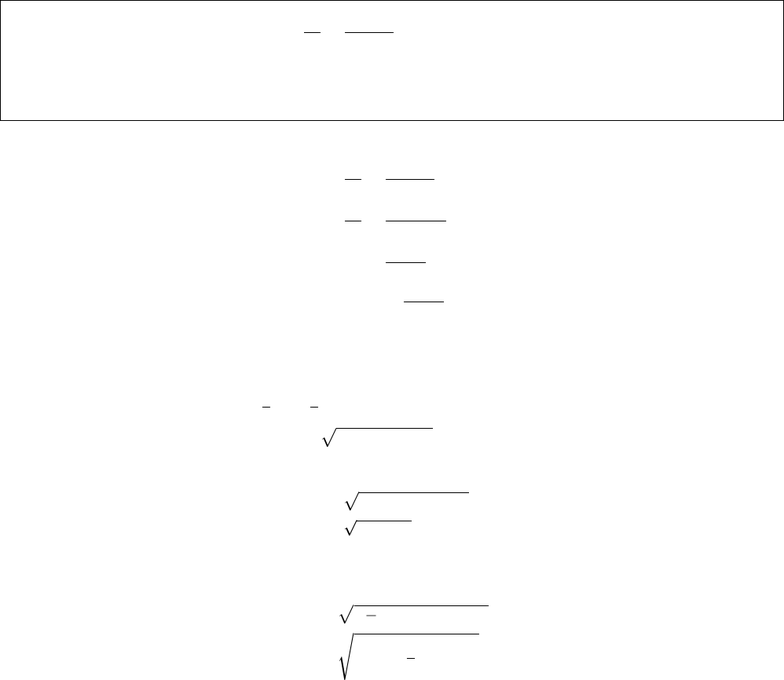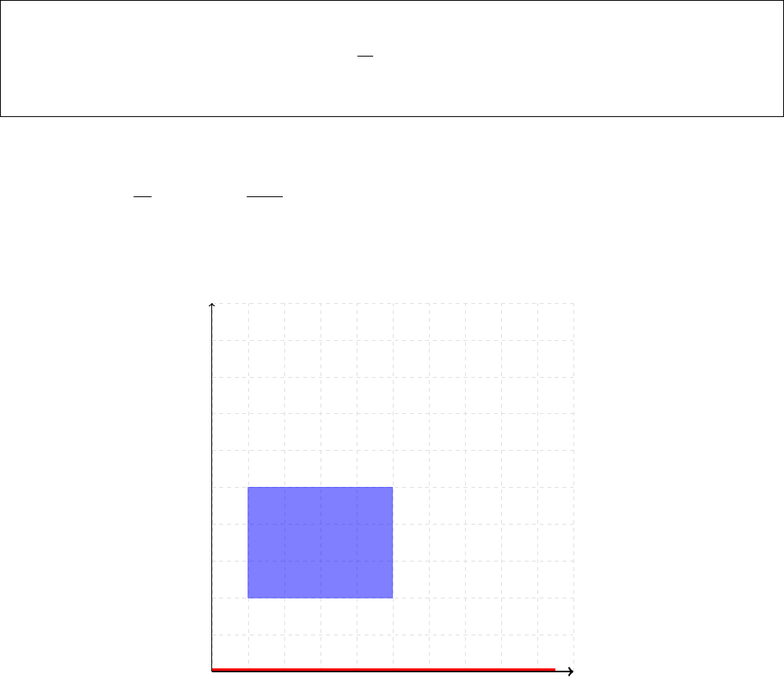Study Guides (400,000)
US (230,000)
CU-Boulder (1,000)
MATH (100)
All (7)
Final

# MATH 3001 Lecture Notes - Lecture 3: Linear Map, Integrating FactorExam

Department
Mathematics
Course Code
MATH 3001
Professor
All
Study Guide
Final

This preview shows pages 1-2. to view the full 6 pages of the document.APPM 2360 Exam 1, Review 1 Solutions
IVPs: Consider the diﬀerential equation dy
dt =t
t2y+y.
(a) Find the general solution for this DE.
(b) Find the solution for this DE that passes though the point (1,2).
(a) First, we may separate variables and integrate
dy
dt =t
t2y+y,
dy
dt =t
y(t2+ 1),
y dy =t
t2+ 1 dt,
Zy dy =Zt
t2+ 1 dt.
Integrating yields
1
2y2=1
2ln |t2+ 1|+c,
y(t) = ±pC+ ln(t2+ 1), C = 2c.
(b) We have an initial condition y(1) = 2, so we take the positive solution from above:
2 = pC+ ln [(1)2+ 1],
2 = C+ ln 2,
C= 4 ln 2.
Substituting back into the general solution gives
y(t) = p4ln 2 + ln(t2+ 1),
=s4 + ln 1
2(t2+ 1)

Only pages 1-2 are available for preview. Some parts have been intentionally blurred.Picard’s Theorem: T/F + Explanation: Both conditions of Picard’s Theorem hold for the following IVP:
dv
dt =kv2/3,
v(3) = 4.
If we treat this DE as v=f(t, v), we have f(t, v) = kv2/3. This function is continuous for all values of tand
v, and so Picard’s Theorem guarantees that a solution exists for any initial condition.
Considering f
v =fv(t, v) = 2k
3v1/3, we see that this function is discontinuous at v= 0. However, given our
initial condition v(3) = 4, it is possible to draw a rectangle around the IC such that fyis continous everywhere in
the rectangle.
(In other words, we can draw a rectangle that doesn’t intersect v= 0).
t
v